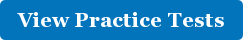## ISEE Mathematics Achievement

• Posted By: Nora Martin
• July 23, 2014The Mathematics Achievement (MA) section of the ISEE Test is intended to reflect curriculum related questions, in contrast to the Quantitative Reasoning section which is intended to measure students’ reasoning abilities. While many students struggle more with the Quantitative Reasoning math section, some students find the Mathematics Achievement section to be very difficult, especially because it is the sections with the most questions per allotted time.
ISEE Level # of questions Minutes to complete section Average Time per question
Lower Level ISEE 30 30 1 minute
Middle Level ISEE 47 40 51 seconds
Upper Level ISEE 47 40 51 seconds

Question type(s): All of the MA questions are standard multiple-choice questions. Subtleties:
• Students often need to learn math concepts they haven’t seen yet to succeed on this section.
• This is the last section before the essay, so students tend to be fatigued by this point in the exam.
Example Lower Level ISEE problem:
What is the name of a rectangle with sides of equal length? (A) hexagon (B) octagon (C) pentagon (D) square
Notice that this is a question that tests curriculum knowledge. Students either know or don't know the definition of a square. Example Middle Level ISEE problem:
The surface area of a cube is 216 square feet. What is the volume of the cube? (A) 6 cubic feet (B) 36 cubic feet (C) 54 cubic feet (D) 216 cubic feet
Notice that to solve this problem, a student must know how to find the area of a cube as well as how to find the volume of a cube. Example Upper Level ISEE problem:
Which type of number could NOT result from the difference of two irrational numbers? (A) integer (B) rational number (C) complex number (D) irrational number
In order to solve this problem, it is helpful for a student to know what both irrational and complex numbers are.

### Find all of our ISEE practice tests and preparation materials at www.ISEEpracticetest.com